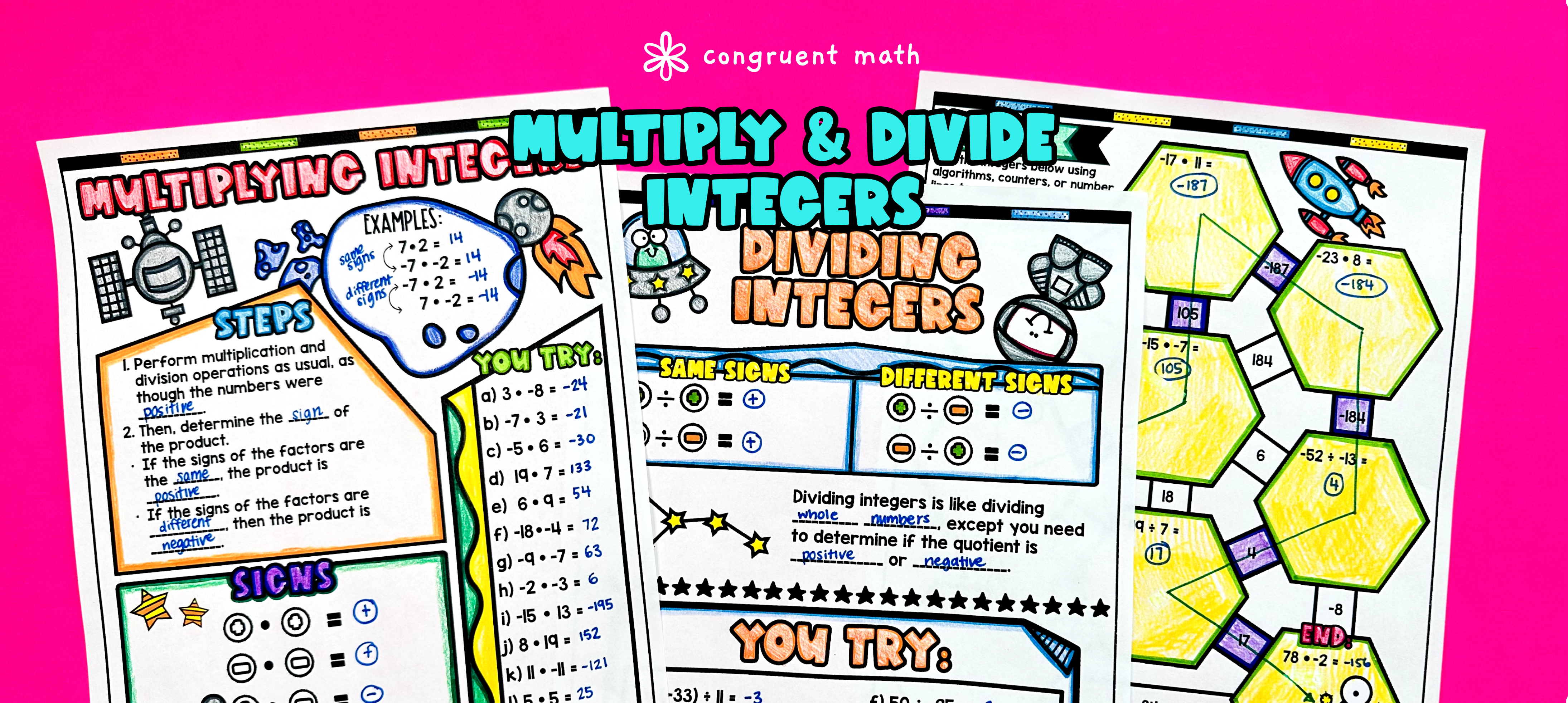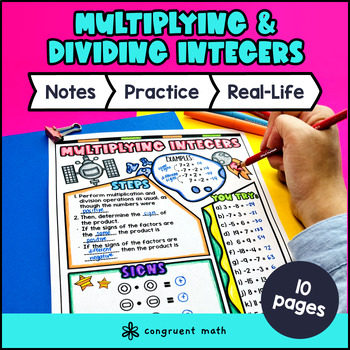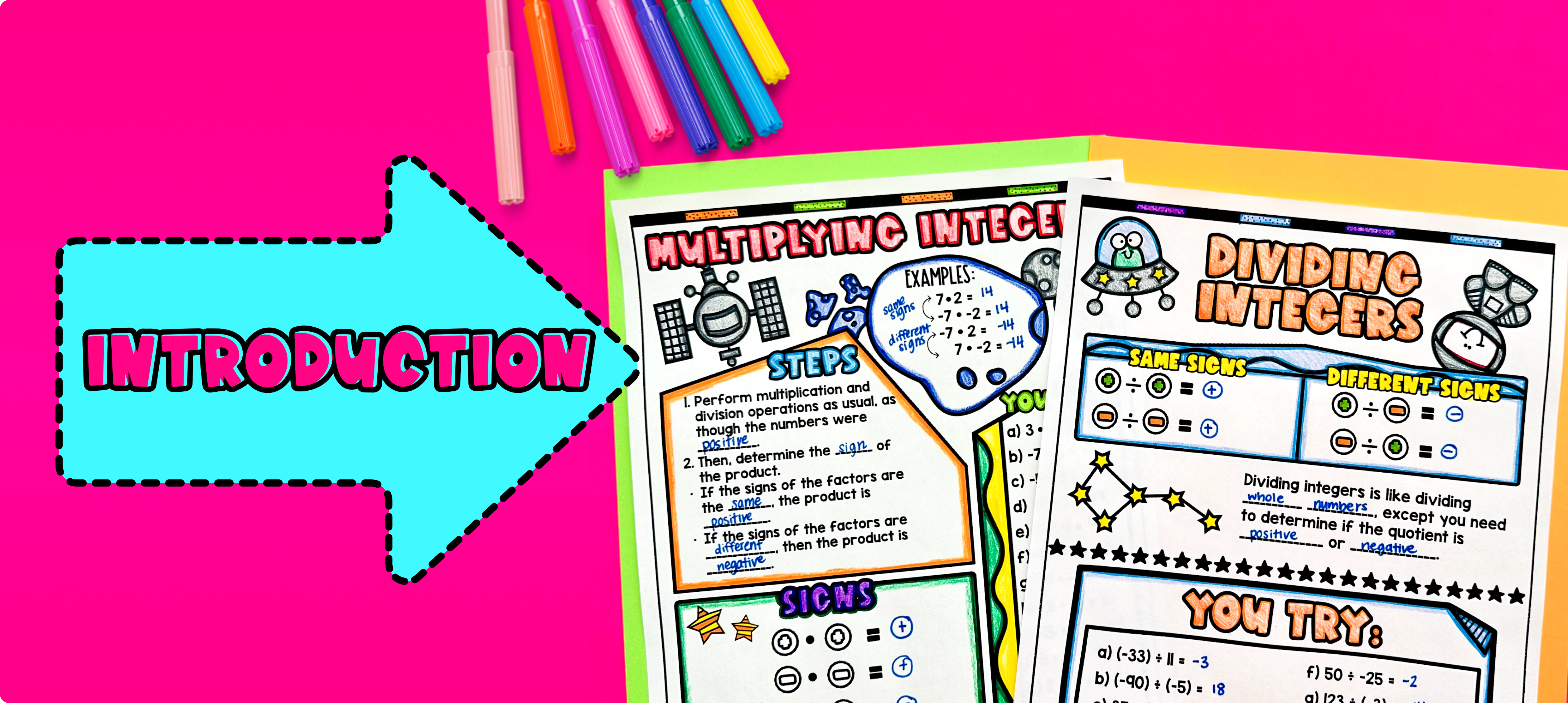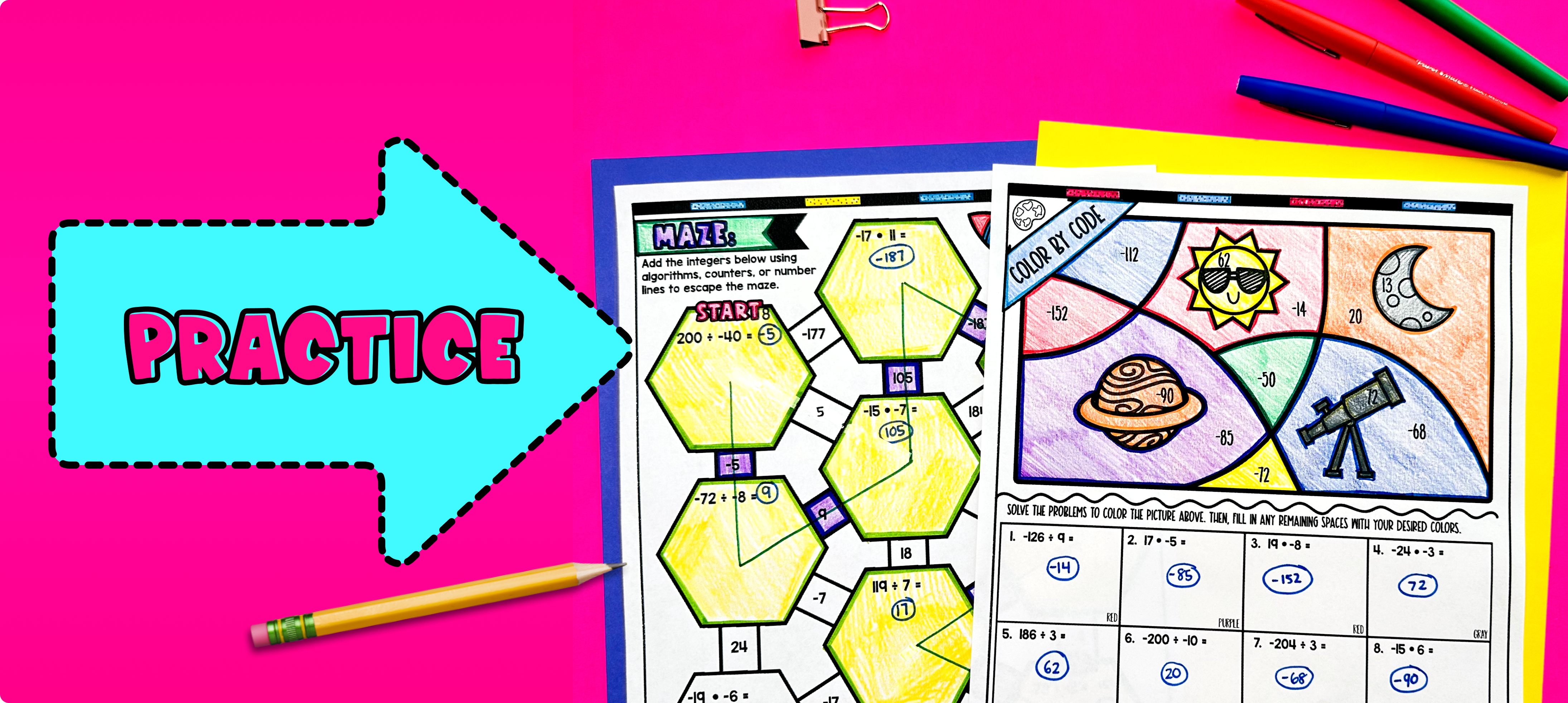# Multiplying and Dividing Integers Lesson PlanPin This

## Overview

Ever wondered how to teach multiplying and dividing integers in an engaging way to your 7th and 8th grade math students?

In this lesson plan, students will learn about integer multiplication and division and their real-life applications. Through artistic, interactive guided notes, checks for understanding, a doodle and color by number activity, and a maze worksheet, students will gain a comprehensive understanding of multiplying and dividing integers.

The lesson culminates with a real-life example that explores how this math concept can be applied to predicting future cost or revenues.

## Get the Lesson Materials\$4.25

## Learning Objectives

After this lesson, students will be able to:

• Multiply integers, both positive and negative

• Divide integers, both positive and negative

• Apply multiplication and division of integers to solve real-life problems

## Prerequisites

Before this lesson, students should be familiar with:

• Understanding of what positive and negative integers are

• Multiplying and dividing whole numbers

## Key Vocabulary

• Integers

• Multiplication

• Division

• Positive

• Negative

## Procedure

### IntroductionPin This

As a hook, ask students why it is important to understand how to multiply and divide integers in real-life situations. You can provide examples such as calculating gains or losses in financial transactions, or finding the total cost of purchases. Refer to the last page of the guided notes as well as the FAQs below for more ideas.

Use the first page of the guided notes to introduce the concept of multiplying integers. Walk through the key points of multiplying positive and negative integers, emphasizing the rules and patterns involved. Explain the concept of a positive number times a positive number resulting in a positive product, a negative number times a negative number resulting in a positive product, and a positive number times a negative number resulting in a negative product. Use examples and visual representations to reinforce the concept. Refer to the FAQ below for a walk through on this, as well as ideas on how to respond to common student questions.

Use the second page of the guided notes to introduce the concept of dividing integers. Walk through the key points of dividing positive and negative integers, highlighting the rules and patterns. Emphasize the concept of a positive number divided by a positive number resulting in a positive quotient, a negative number divided by a negative number resulting in a positive quotient, and a positive number divided by a negative number resulting in a negative quotient. Provide examples and visual aids to help students grasp the concept. Refer to the FAQ below for a walk through on this, as well as ideas on how to respond to common student questions.

Based on student responses, reteach concepts that students need extra help with. If your class has a wide range of proficiency levels, you can pull out students for reteaching, and have more advanced students begin work on the practice exercises.

### PracticePin This

Have students practice multiplying and dividing integers using the maze activity. Walk around to answer student questions.

Fast finishers can dive into the color by number activity for extra practice. You can assign it as homework for the remainder of the class.

### Real-Life ApplicationPin This

Bring the class back together, and introduce the concept of predicting future cost or revenues using multiplying and dividing integers. Explain that this concept can be applied in real life situations such as budget planning and financial projects.

To illustrate this concept, provide the students with a scenario. For example, imagine a local business owner who wants to predict their future monthly revenue based on their past performance.

Ask the students to think about what factors could affect the business's revenue. Discuss with the class possible factors such as seasonality, economic conditions, marketing efforts, and customer trends. Next, explain that the business owner has collected data on their monthly revenue over the past year and wants to use this data to forecast their revenue for the next year.

Using the guided notes and the concepts they have learned about multiplying and dividing integers, instruct the students to brainstorm other scenarios in real life where multiplying and dividing integers can be used.

Finally, facilitate a class discussion on how the business owner could use this forecasted revenue to make informed decisions about budgeting, marketing strategies, and resource allocation. Encourage the students to think critically about the importance of understanding and applying integer operations in real-life situations.

Refer to the FAQ section of the lesson plan for more ideas and examples on how to teach real-life applications of multiplying and dividing integers.